### Basic Geometrical Ideas-Solutions Ex-4.2

CBSE Class –VI Mathematics
NCERT Solutions
Chapter 4
Basic Geometrical Ideas (Ex. 4.2)

Question 1. Classify the following curves as (i) Open or (ii) Closed.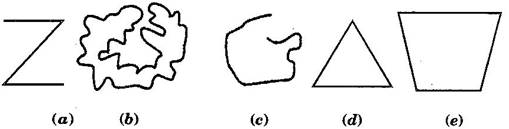(b) Closed curve
(c) Open curve
(d) Closed curve
(e) Closed curve
Question 2. Draw rough diagrams to illustrate the following:
(a) Open curve
(b) Closed curve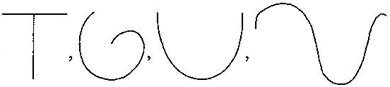Closed curves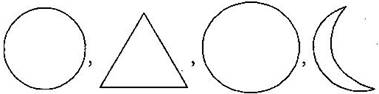Question 3. Draw any polygon and shade its interior.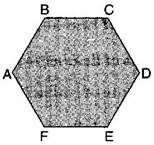Question 4. Consider the given figure and answer the questions:
(a) Is it a curve?
(b) Is it closed?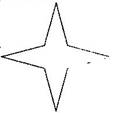Answer: (a) No, it is not a curve.
(b) No, it is not closed.
Question 5. Illustrate, if possible, each one of the following with a rough diagram:
(a) A closed curve that is not a polygon.
(b) An open curve made up entirely of line segments.
(c) A polygon with two sides.
Answer: (a)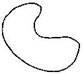(b)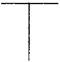(c) Polygon with two sides cannot be draw.